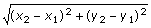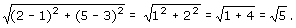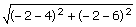## Distance Formula

Definition of Distance Formula

The formula, which is used to find the distance between two points (x1y1) and (x2y2), is called the Distance Formula.

### Examples of Distance Formula

The distance between the points (1, 3) and (2, 5) isSolved Example on Distance Formula

What is the distance between the points A (4, 6) and B (- 2, - 2)?
Choices:
A. 10
B. 6
C. 8
D. 4

Step 2: Distance between the two points can be found using the distance formula.
Step 3: So, AB == 10 units.